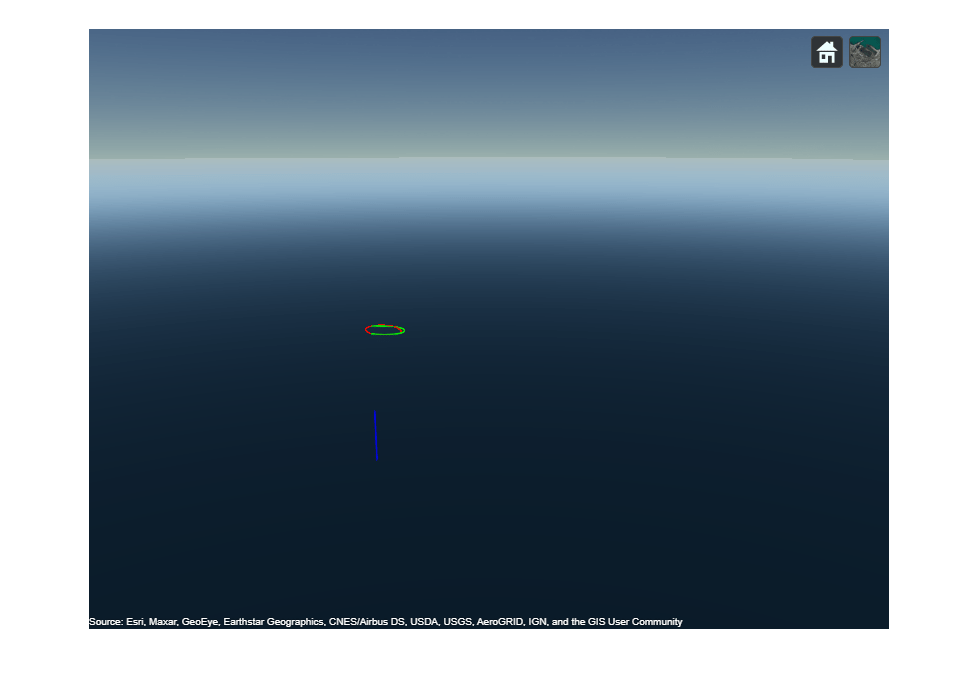# plotTrajectory

Plot trajectories in `trackingGlobeViewer`

## Syntax

``plotTrajectory(viewer,trajectories)``
``plotTrajectory(___,Name,Value)``

## Description

````plotTrajectory(viewer,trajectories)` plots trajectories on the tracking globe viewer.```

example

````plotTrajectory(___,Name,Value)` specifies options using one or more name-value pair arguments. For example, `plotTrajectory(viewer,trajectories,Color=[1 0 0])` specifies the color of the plotted trajectory as the RGB triplet `[1 0 0]`.```

## Examples

collapse all

Create a tracking globe viewer and specify its reference location and camera view.

```refloc = [1 -5 50]; viewer = trackingGlobeViewer(ReferenceLocation=refloc); campos(viewer,refloc + [-0.02 0 1000]); camorient(viewer,[10 -15 0]);```

Create three trajectories.

• The first trajectory is a waypoint trajectory.

• The second trajectory is also a waypoint trajectory, but its reference frame is specified as an ENU frame instead of the default NED frame.

• The third trajectory is a `geoTrajectory`, which specifies waypoints in geodetic coordinates.

```traj1 = waypointTrajectory([0 0 -400; 100 0 -400; 0 100 -400],[0 200 400]); traj2 = waypointTrajectory([0 0 400; 100 0 400; 0 100 400],[0 200 400],ReferenceFrame="ENU"); traj3 = geoTrajectory([1 -5 0; 1.001 -5 0; 1.002 -5 100],[0 3600 7200]);```

Plot the three trajectories one by one.

```plotTrajectory(viewer,traj1,Color=[1 0 0]); plotTrajectory(viewer,traj2,Color=[0 1 0]); plotTrajectory(viewer,traj3,Color=[0 0 1]);```

Take a snapshot and show the results.

```drawnow snapshot(viewer)```## Input Arguments

collapse all

Tracking globe viewer, specified as a `trackingGlobeViewer` object.1

Target trajectories, specified as a `waypointTrajectory` object, a `geoTrajectory` object, or a cell array of `waypointTrajectory` objects or `geoTrajectory` objects. When specified as a cell array, trajectories can contain both `waypointTrajectory` objects and `geoTrajectory` objects. The reference frame used to plot the `waypointTrajectory` object is the local NED or ENU frame with its origin specified by the `ReferenceLocation` property of the `viewer`.

### Name-Value Arguments

Specify optional pairs of arguments as `Name1=Value1,...,NameN=ValueN`, where `Name` is the argument name and `Value` is the corresponding value. Name-value arguments must appear after other arguments, but the order of the pairs does not matter.

Example: ```plotTrajectory(viewer,trajectories,Color=[1 0 0])```

Width of the trajectory line, specified as a positive value in points, where 1 point = 1/72 of an inch. The line width cannot be thinner than the width of a pixel. If you set the line width to a value that is less than the width of a pixel on your system, the line displays as one pixel wide.

Color of trajectories, specified as one of these options:

• A 1-by-3 RGB triplet — Plot all the trajectories with the same color.

• An N-by-3 matrix of RGB triplets — Plot each trajectory in a different color, where N is the number of trajectories.

## Version History

Introduced in R2021b